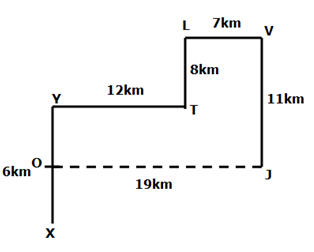# NIACL AO Prelims – Reasoning Ability Questions 2021 (Day-03)

Dear Aspirants, Our IBPS Guide team is providing new series of Reasoning Questions for NIACL AO Prelims 2021 so the aspirants can practice it on a daily basis. These questions are framed by our skilled experts after understanding your needs thoroughly. Aspirants can practice these new series questions daily to familiarize with the exact exam pattern and make your preparation effective.

Start Quiz

Floor based puzzle

Direction (1-5): Study the following information carefully and answer the questions given below.

Eight persons A, B, C, D, E, F, G, and H are staying in a building of eight floors but not necessarily in the same order. The ground floor is numbered as 1, the floor just above the ground floor is numbered as 2, and so on till the topmost floor is numbered as 8.

E stays on the 4th floor. Two persons stay between E and D. G stays adjacent to D. Four persons stay between G and C, who doesn’t stays above E. Only one person stays between G and B. As many persons stay above A is one less than the persons who stay below A. H stays neither at the bottom nor adjacent to C.

1) Who among the following person stays on the ground floor?

A.The one who stays two floors below H

B.F

C.The one who stays immediately below E

D.B

E.None of these

2) How many persons stay between F and G?

A.As many persons stay between B and A

B.One

C.Three

D.As many persons stay above E

E.None

3) Four of the following five are alike in a certain way and thus form a group as per the given arrangement. Which of the following does not belong to that group?

A.D

B.A

C.H

D.C

E.B

4) If all the persons are arranged in alphabetical order from top to bottom, then how many persons remain in the same position?

A.One

B.Two

C.Three

D.Four

E.No one

5) If C is related to B and E is related to A, then who among the following person is related to G?

A.H

B.F

C.D

D.B

E.None of these

Inequality

Direction (6-8): In each of the following questions, the relationship between different elements is shown in the statements followed by two conclusions. Find the conclusion which is logically follows.

6) Statements:

N ≤ G < V > Q; S ≥ P > Q > L; Z < I = S

Conclusions:

I)I > L

II)G < P

A.If only conclusion I follows.

B.If only conclusion II follows.

C.If either conclusion I or II follows.

D.If neither conclusion I nor II follows.

E.If both conclusions I and II follow.

9) Statements:

M < V ≤ P < O; Y > X ≥ O; X > R ≥ E  >Q;

Conclusions:

I)V ≤ X

II)E < Y

A.If only conclusion I follows.

B.If only conclusion II follows.

C.If either conclusion I or II follows.

D.If neither conclusion I nor II follows.

E.If both conclusions I and II follow.

8) Statements:

H < Q < M ≤ P; H > I < J ≥ L; V > Y ≤ N = P

Conclusions:

I) Y > Q

II) J > M

A.If only conclusion I follow.

B.If only conclusion II follows.

C.If either conclusion I or II follows.

D.If neither conclusion I nor II follows.

E.If both conclusions I and II follow.

Direction sense

Direction (9-10): Study the following information carefully and answer the below questions

One fine morning, Samyutha’s shadow falls towards her left and she starts walking from Point X for 6km to reach point Y where she turns to the right and walks for 12km to reach point T. Then she turns to the left and walks for 8km to reach point L. Then she turns two consecutive rights for 7km and 11 km respectively to reach point V and Point J respectively.

9) If Point O is exactly in the middle of Point Y and X, then what is the direction and distance of O with respect to Point J?

A.19km towards east

B.19km towards west

C.11km towards west

D.12km towards east

E.Can’t be determined

10) What is the direction of Point L with respect to Point X?

A.East

B.North-West

C.North-East

D.South

E.South-west

### Try NIACL AO Online Practice Mock Test

Directions (1-5) :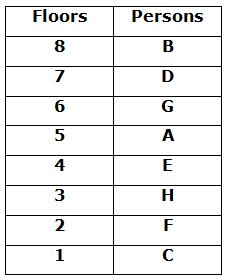We have,

• E stays on the 4th
• Two persons stay between E and D.
• G stays adjacent to D.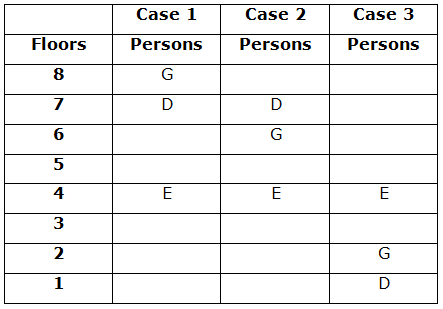Again we have,

• Four persons stay between G and C, who doesn’t stays above E.
• Only one person stays between G and B.So case-3 gets eliminated.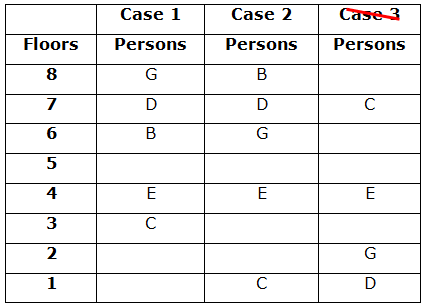Again we have,

• As many persons stay above A is one less than the persons who stay below A.
• H stays neither at the bottom nor adjacent to C. so case-1 gets eliminated.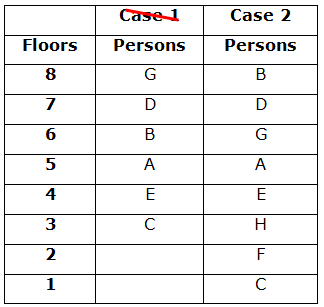I) I > L (I = S ≥ P > Q > L) –> True

II) G < P (G < V > Q < P) –> False

Only conclusion I follow

I) V ≤ X (V ≤ P < O ≤ X) –> False

II) E < Y (Y > X > R ≥ E) –> True

Only conclusion II follows.

I) Y > Q (Q < M ≤ P = N ≥ Y) –> False

II) J > M(M > Q > H > I < J) –> False

Neither conclusion I nor II follows.

Directions (9-10) :